NCERT Solutions for Class 10 Mathematics Chapter 3 Pair of Linear Equations in Two Variables Ex 3.2 are part of NCERT Solutions for Class 10 Maths. Here we have given NCERT Solutions for Class 10 Mathematics Chapter 3 Pair of Linear Equations in Two Variables Ex 3.2.

 Board CBSE Textbook NCERT Class Class 10 Subject Maths Chapter Chapter 3 Chapter Name Pair of Linear Equations in Two Variables Exercise Ex 3.2 Number of Questions Solved 7 Category NCERT Solutions

### NCERT Solutions for Class 10 Maths Chapter 3 Pair of Linear Equations in Two Variables Ex 3.2

Class 10th Exercise 3.2 Question 1.
Form the pair of linear equations in the following problems, and find their solutions graphically.
(i) 10 students of class X took part in a Mathematics quiz. If the number of girls is 4 more than the number of boys, find the number of boys and girls who took part in the quiz.
(ii) 5 pencils and 7 pens together cost ₹ 50, whereas 7 pencils and 5 pens together cost ₹ 46. Find the cost of one pencil and that of one pen.
Solution:
(i) Let the number of girls be x and number of boys be y.
A.T.Q.
1st Condition :
x +y =10
Table :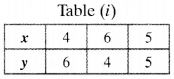2nd Condition :
x = y + 4 ⇒ x – y = 4
Table :Solving
(i) and
(ii) graphically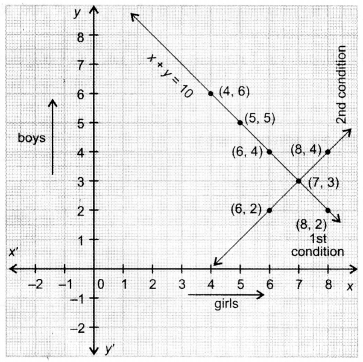Both the lines cut at (7, 3)
Hence, solutions is (7, 3), i.e.x = 7,y = 3
Number of girls – 7 and Number of boys = 3

(ii) Let cost of 1 pencil = ₹ x and cost of 1 pen = ₹ y.
A.T.Q.
1st Condition :
5x + 7y = 50
Table :2nd Condition :
7x + 5y = 46
Table :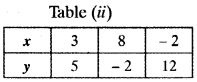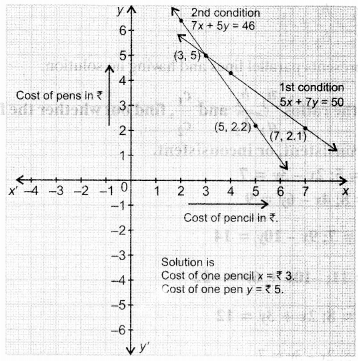Class 10 Maths Ex 3.2 Question 2.
On comparing the ratios and find out whether the lines representing the following pairs of linear equations intersect at a point, are parallel or coincident:
(i) 5x – 4y + 8 = 7x + 6y – 9 = 0
(ii) 9x + 3y + 12 = 0, 18x + 6y + 24 = 0
(iii) 6 – 3y + 10 = 0, Zx – v + 9 = 0
Solution:
(i) Equations are 5x – 4y + 8 = 7x + 6y – 9 = 0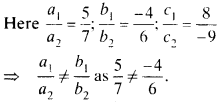∴ Pair of lines represented by given equations intersect at one point. So, the system has exactly one solution.

(ii)
9x + 3y + 12 = 0, 18 + 6y + 24 = 0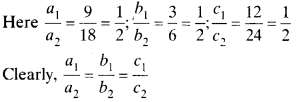∴ Pair of equations represents coincident lines and having infinitely many solutions.

(iii)
6x – 3y + 10 = 0, 2x -y + 9 = 0∴ It represents parallel lines and having no solution.

Ex 3.2 NCERT Class 10 Question 3.
On comparing the ratios and find out whether the following pair of linear equations are consistent, or inconsistent,

(i) 3x + 2y = 5; 2x – 3y = 7
(ii) 2x – 3y = 8; 4x – 6y = 9
(iii) 3/2x + 5/3y = 7; 9x – 10y = 14
(iv) 5x-3y = 11; -10c + 6y = -22
(v) 4/3x + 2y = 8; 2x + 3y = 12

Solution: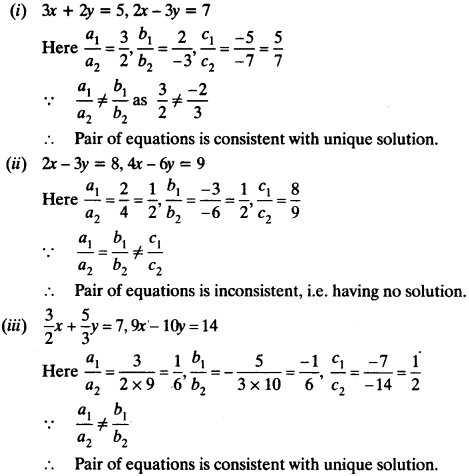∴ Pair of equations is consistent with infinitely many solutions.

Ex 3.2 Class 10 NCERT Solutions Question 4.
Which of the following pairs of linear equations are consistent/inconsistent If consistent, obtain the solution graphically:
(i) x + y = 5, 2x + 2y = 10
(ii) x – y = 8, 3x – 3y = 16
(iii) 2x + y – 6 = 0, 4x – 2y – 4 = 0
(iv) 2x – 2y – 2 = 0, 4x – 4y – 5 = 0

Solution:
(i) x + y – 5, 2x + 2y = 10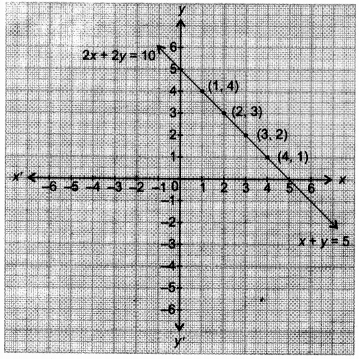Here,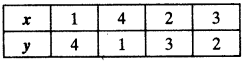∴ System of equations is consistent and the graph gr represents coincident lines.
Table for equation (i),Table for equation (ii),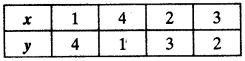(ii) x – y = 8, 3x – 3y = 16
Here $$\frac { a_{ 1 } }{ a_{ 2 } } =\frac { 1 }{ 3 } ,$$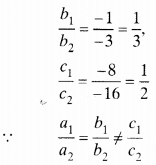Pair of equations is inconsistent. Hence, lines are parallel and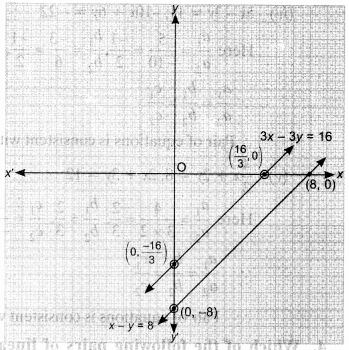(iii)
2x + y – 6 = 0, 4x – 2y – 4 = 0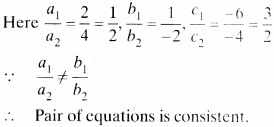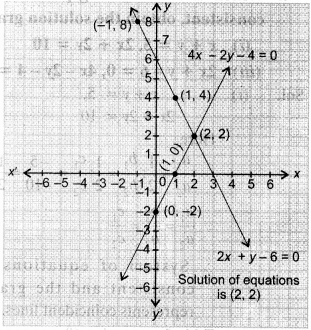Pair of equations is consistent.
Table for equation 2x + y – 6 = ()Table for equation 4x – 2y – 4 = 0(iv)
2x – 2y – 2 = 0, 4x – 4y – 5 = 0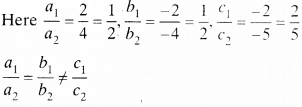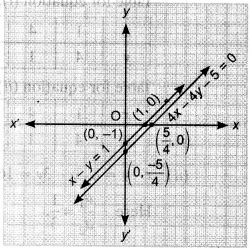∴ Pair of equations is inconsistent. Hence, lines are parallel and system has no solution.

Class 10 Maths Chapter 3 Exercise 3.2 Question 5.
Half the perimeter of a rectangular garden, whose length is 4 m more than its width, is 36 m. Find the dimensions of the garden.
Solution:
Let length of garden = x m and width of garden = y m
Perimeter of rectangular garden = 2(x + y)
A.T.Q.
1st Condition :
$$\frac { 2(x+y) }{ 2 }$$ = 36 ⇒ x + y = 36
2nd Condition :
x = y + 4 ⇒ x -y = 4 … (ii)
Adding equation (i) and (ii), we get
2x = 40 ⇒ x = 20
Putting x = 20 in equation (i), we get
20 + y = 36
y = 16
Hence, dimensions of the garden are 20 m and 16 m.

Exercise 3.2 Class 10 Question 6.
Given the linear equation 2x + 3y – 8 = 0, write another linear equation in two variables, such that the geometrical representation of the pair so formed is:
(i) intersecting lines
(ii) parallel lines
(iii) coincident lines
Solution:
Given equation is 2x + 3y – 8 – 0
We have 2x + 3y = 8
Let required equation be ax + by = c
Condition :
(i) For intersecting lines$$\frac { 2 }{ a } \neq \frac { 3 }{ b } \neq \frac { 8 }{ c }$$ where a, b, c can have any value which satisfy the above condition.
Let a = 3, b = 2, c = 4
so, $$\frac { 2 }{ 3 } \neq \frac { 3 }{ 2 } \neq \frac { 8 }{ 4 }$$
∴ Equations are 2v + 3y = 8 and 3A + 2y = 4 have unique solution and their geometrical representation shows intersecting lines.

(ii) Given equation is 2x + 3y = 8 Required equation be ax + by = c
Condition :
For parallel lines
$$\frac { 2 }{ a } \neq \frac { 3 }{ b } \neq \frac { 8 }{ c }$$
where a, b, c can have any value which satisfy the above condition.
Let, a = 2, b = 3, c = 4
Required equation will be 2x + 3y = 4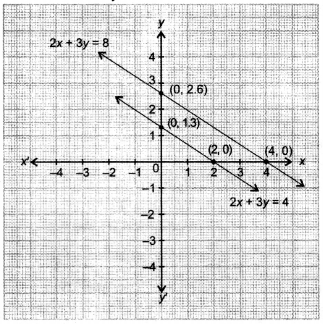Equations 2x + 3y = 8 and 2x + 3y = 4 have no solution and their geometrical representation shows parallel lines.

(iii)
For coincident lines: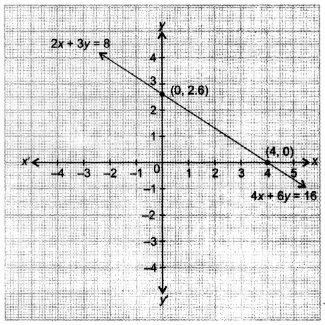Given equation is 2x + 3y = 8 Let required equation be ax + by = c For coincident lines.
$$\frac { 2 }{ 3 } =\frac { 3 }{ 2 } =\frac { 8 }{ 4 }$$ where a, b, c can have any a b c possible value which satisfy the above condition.
Let a = 4, b = 6, c = 16 Required equation will be 4x + 6y = 16.
Equations 2x + 3y = 8 and 4x + 6y = 16 have infinitely many solutions and their geometrical representation shows coincident lines.

NCERT Ex 3.2 Class 10 Question 7.
Draw the graphs of the equations x – y + 1 = 0 and 3x + 2y -12 = 0. Determine the coordinates of the vertices of the triangle formed by these lines and the x – axis, and shade the triangular region.
Solution:
First equation is x – y + 1 = 0.
Table for 1st equation x = y – 1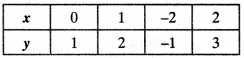Second equation is 3x + 2y = 12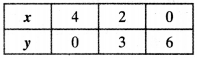⇒ 3x = 12 – 2y ⇒ x = $$x=\frac { 12-2y }{ 3 }$$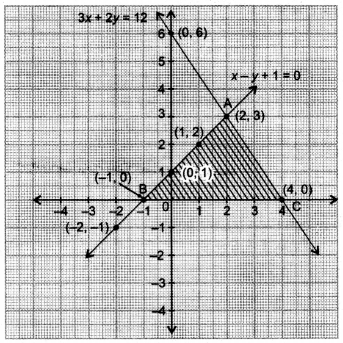Required triangle is ABC. Coordinates of its vertices are A(2, 3), B(-1, 0), C(4, 0).

We hope the NCERT Solutions for Class 10 Mathematics Chapter 3 Pair of Linear Equations in Two Variables Ex 3.2 help you. If you have any query regarding NCERT Solutions for Class 10 Mathematics Chapter 3 Pair of Linear Equations in Two Variables Ex 3.2, drop a comment below and we will get back to you at the earliest.